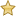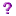Lecture 6 - MIPS, OS, Bit-Level Operations

Thursday, June 19, 2014

4:46 PM

Example

 Main:                lis \$2 .word 13 add \$3, \$0, \$0 top:                add \$3, \$3, \$2 lis \$1 .word 1 sub \$2, \$2, \$1 bne \$2, \$0, top jr \$31 beyond:

Pass 1: Group tokens into instructions. Build symbol table:

 label Address main top beyond 0x00 0x0c 0x24

Pass 2: Translate each instruction:

eg: lis \$2 -> 0x00001014

bne \$2, \$0, top -> lookup top in symbol: 0x0c

calculate (top – PC)/4 = -5

-> ox1440fffb (-5 = 0xfffb)

To negate a 3’s complement number, flip the bits and add 1Bit-level Operations

To assemble bne \$2, \$0, top (where (top-PC)/4 = -5).

opcode = 000101 = 5

first register = 2 = 00010

second register = 0 = 00000

offset = -5

To put 000101 in the first 6 bits, need to append 26 0’s, i.e. left-shift by 26 bits.

C/C++: 5 << 26

Racket: (arithmetic-shift 5 –26)

Move \$2 21 bits to the left.

Move \$0 15 bits to the left.

-5 = 0xfffffffb, which is 32 bits. there is only room for 16 bits.

So bitwise and with 0xffff

C: -5 & 0xffff

Racket: (bitwise-and -5 #^ffff)

result: 0xfffb

Then bitwise-or the 4 pieces together.

instr = (5 << 26) | (2 << 21) | (0 << 16) | (-5 & 0xffff)

cout << instr;

This will not work. Will print out: 339 804 155

this is 72 bits. Each digit became ASCII

Solution:

 char c = instr        ;x – last 8 bits of instr char c = instr >> 24; cout << c; c = instr >> 16; cout << c; c = instr >> 8; cout << c; c = instr; cout << c;Loaders

OS code:

 repeat: P <- next program to run copy P into memory, starting at 0x00    ;loader jalr \$0 beq \$0, \$0, repeat

Problems:OS is also a program – where does it sit in memory?

Are there other programs? – they can’t all be at address 0x00

How to fix this: choose different starting address for programs at assembly time

More problems:How will the loaders know where to load them?What if two of the same programs are running?

Loader’s job – take program P as input

find a location α in memory for P

copy P to this location

return α to OS

OS 2.0

 repeat: P <- next program to run \$3 <- loader (P) jalr \$3 beq \$0, \$0, repeat

 Input: words w1,...,wk (machine code for P) n = k + space for stack <- how much stack space? (pick something!) α = first address of n contiguous words of free RAM for i = 1..k MEM[α+(i-1)*4] <- wi \$30 <- α+4*n return α

Problem: We can’t just load programs anywhere. Labels may resolve to wrong addresses.

loader will have to fix these somehow.

What needs to change when we relocate?

• .word id                        <- add α to id
• .word constant                <- do NOT relocate
• everything else         <- do NOT relocate

Created with Microsoft OneNote 2010
One place for all your notes and information